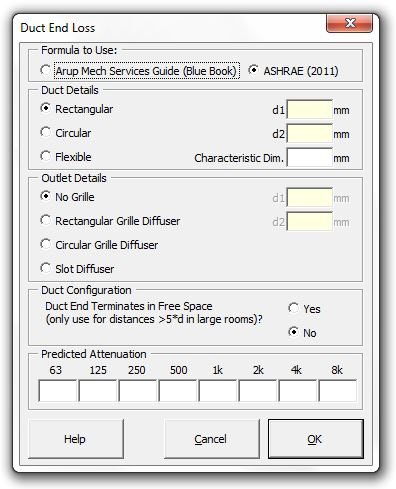### Strutt Help

Duct End Loss    1/1

Strutt|Mechanical Services|Duct Elements|End/Grille inserts the attenuation due to duct end reflection into the active row of the worksheet.Attenuation due to duct end reflection can be calculated using the referenced values in the Arup Sound and Vibration in Mechanical Services Design (Blue Book) or the ASHRAE (2011) method.

Blue Book method references values in Table 17, where the duct dimension referred to is either the diameter of circular ducts or 1.13 * A^2, where A is the section area of rectangular ducts. For grilles this is the core area of the grille and for ceiling diffusers it is 1.25 * D, where D is the neck diameter.

The ASHRAE 2011 method is based on more-recent research from 2008 and is considered more-accurate.
Strutt calculates the attenuation associated with duct-end reflection losses from sheet-metal ductwork as follows:

• No grille/termination:
• Delta L_"no grille" = -10log_10 [1 + ((a_1 c_0)/(pi f D))^(a_2)]
Coefficients a_1 and a_2 vary depending on whether the duct termination is in free space or flush with a surface. Note that research shows that 'free space' terminations are rare in practice (the duct should be ~5D or more from any surface - including a tiled ceiling - for 'free space' conditions to exist).
• Where the duct terminates with a grille the end reflection loss is reduced by 2-5 dB. Strutt accounts for this as follows:
Delta L = Delta L_"no grille" -3 where Delta L_"no grille" >= 4
Delta L = (Delta L_"no grille")/3 where Delta L_"no grille" <4
• Where the duct terminates in a narrow aspect slot diffuser, the end reflection loss is proportional to the area change:

Delta L=10log((4 A_(duct) A_("grille"))/(A_(duct)+A_("grille"))^2)
Where:
f is frequency (Hz)
c_0 is the speed of sound in air
D is the diameter of a circular duct of the effective diameter of a rectangular duct

Note that no significant end reflection will occur for flexible ductwork; if flexible ductwork is selected then the end reflection is set to zero.

Reference: Michaud and Cunefare (2008) Experimental Investigation of Reflection of Airborne Noise at Duct Terminations, ASHRAE Research Project RP-1314